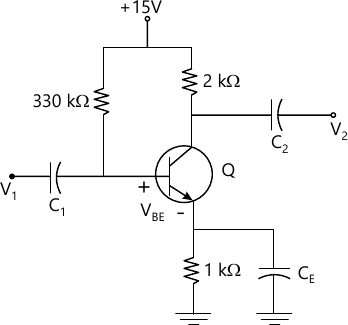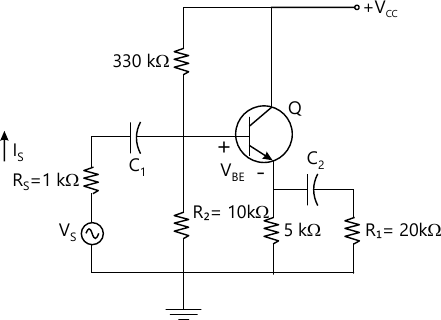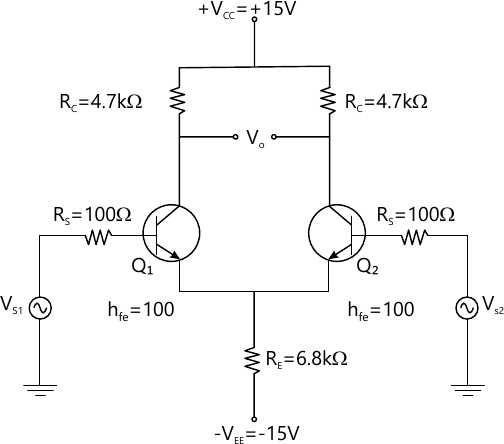MORE IN Analog and Digital Electronics
VTU Computer Science (Semester 3)
Analog and Digital Electronics
December 2011
Total marks: --
Total time: --
INSTRUCTIONS
(1) Assume appropriate data and state your reasons
(2) Marks are given to the right of every question
(3) Draw neat diagrams wherever necessary

1 (a) Discuss with neat sketches, the relation of operating point of transistor for following cases
(i) Neat saturation region
(ii) Neat cut-off region
(iii) At the centre of active region
8 M
1 (b) For the circuit shown in Fig. Q1 (b), calculate IB, IC, VCE, VC, VE, VB and VBC. Assume B=1008 M
1 (c) Explain the basic methods of triggering of SCR.
4 M

2 (a) Explain with neat sketches, the operation, characteristics and parameter of n-channel depletion type MOSFET.
8 M
2 (b) Explain with neat sketches, the operation of JFET along with its characteristics curves.
8 M
2 (c) Discuss merits, demerits and applications of IGBTs.
4 M

3 (a) Discuss the classification of optoelectronics devices, in detail.
6 M
3 (b) Explain with neat diagrams, the principle of operations, characteristics, advantages, disadvantages and applications of a photodiode.
8 M
3 (c) Briefly discuss with necessary diagrams, the basic operation and construction of LED.
6 M

4 (a) Obtain the expression for current gain, input impedence voltage gain, output impedance power gain of a transistor amplifier using complete h-parameter model.
8 M
4 (b)

In the common collector shown in fig. Q4 (b), the trasistor parameters are hic=1.2 k, hfc=-101, hrc=1 and hcc=25 μA/v. Calculate Ri, Ai, Av and Ro for the circuit.8 M
4 (c) Explain common emitter, common collector amplifier along with it?s a.c. equivalent circuit.
4 M

5 (a) Explain the classification of large signal amplifiers as class A, class B, class C and class AB amplifiers.
6 M
5 (b) An emplifier with openloop voltage gain of 1000, delivers 10W of power output at 10% second harmonic distortion when i/p is 10 mV. A 40 dB negative feedback is applied and output power is to remain at 10W. Determine required input signal Vs and second harmonic distortion with feedback.
8 M
6 M

6 (a) Explain with a neat diagram Hartley oscillater and calpits oscillate as LC oscillator.
6 M
6 (b) Explain the various types of multivibrators. Also mention the applications.
6 M
6 (c) Obtain the expression for time period T at the base of transistor, in case of wave shaping circuits.
8 M

7 (a) Explain with a functional block diagram, a typical three terminal IC voltage regulator.
6 M
7 (b) Discuss the limitations of linear voltage regulators.
6 M
7 (c) Briefly discuss power converters in series and parallel connection along with neat diagrams.
8 M

8 (a) Discuss the requirements of a good instrumentation amplifier.
6 M
8 (b)

Fig. Q8(b) shows dual input, balanced output and differential amplifier configuration. Assuming silica transistor with hie=2.8 KΩ calculate; (i) Operating point valves ; (ii) Differential gain; (ii) Common mode gain; (iv) CMRR; (v) Output if Vs1 =70 mV peak to peak at 1 kHz; vi) Vs2=40 mV peak to peak at 1 kHz.10 M
8 (c) Explain the various electrical characteristics of an ap-amp which are generally in the data sheet.
4 M

More question papers from Analog and Digital Electronics